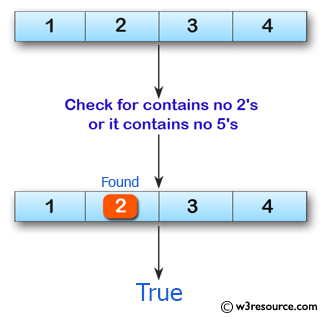﻿ Swift Array Programming Exercise: Check if a given array of integers contains no 2's or it contains no 5's - w3resource# Swift Array Programming Exercises: Check if a given array of integers contains no 2's or it contains no 5's

## Swift Array Programming: Exercise-30 with Solution

Write a Swift program to check if a given array of integers contains no 2's or it contains no 5's.

Pictorial Presentation:Sample Solution:

Swift Code:

``````func no25(array_nums: [Int]) -> Bool {
var num_ctr2 = 0
var num_ctr5 = 0

for num in array_nums {
if num == 2
{
num_ctr2 += 1
}
else if num == 5
{
num_ctr5 += 1
}
}
return num_ctr2 == 0 || num_ctr5 == 0
}

print(no25(array_nums: [1, 4, 3]))
print(no25(array_nums: [1, 2, 3, 4]))
print(no25(array_nums: [1, 3, 5]))
print(no25(array_nums: [2, 5]))
```
```

Sample Output:

```true
true
true
false
```

Swift Programming Code Editor:

Improve this sample solution and post your code through Disqus

What is the difficulty level of this exercise?

﻿